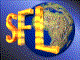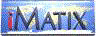| iMatix home page | << | < | > | >>SFLVersion 2.10

### tree_find_le

```#include "sfltree.h"
void *tree_find_le (TREE **root, void *tree, TREE_COMPARE *comp)
```

#### Synopsis

Finds node with data less than or equal to that provided.

#### Source Code - (sfltree.c)

```{
TREE
*current = *root,
*found;

found = NULL;
while (current != TREE_NULL)
switch ((comp) (current, tree))
{
case 1 : current = current-> left;  break;
default: found = current;
current = current-> right;
}

return found;
}
```

 | << | < | > | >>Copyright © 1996-99 iMatix Corporation